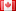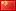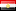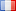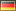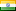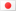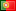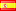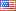Details on the calculators used on DÉCOR PLANit

1. These pages and calculators created with the following technologies:
• HTML
• CSS - Cascading Style sheets
• Javascript - note: Javascript must be enabled to have these calculators function
• JQuery - JavaScript Library
• autoNumeric© - a jQuery plugin that automatically formats numbers as you type to many International standards
• PHP - for server scripts and cross domain queries

2. The following metric/imperial conversion factors are used:
• 1 linear meter = 1.09361329833770781761 linear yards
• 1 linear millimeter = 0.03937007874015747977 inch
• 1 linear micron = 0.03937007874015747977 mil or caliper
• 1 square meter = 10.76391041670972192890 square feet
• 1 Kilogram = 2.20462262184877566540 pounds (USA)

3. Grammage and Basis Weight conversions are done with the following formulas:
• Grammage = (((Basis Weight / lbs per kilo ) / (3000 / ft2 per m2 )) * 1000)
• Basis Weight = ((((Grammage / 1000) * pounds) / square feet) * 3000)
• ft2 per m2 = 10.76391041670972192890
• lbs per kilo = 2.20462262184877566540

4. Numbers are rounded using the "Round-Half-Up (Arithmetic Rounding) symmetric". With any rounding method errors can and do occur.

5. These calculators were tested in the following browsers
1. Internet Explorer 6, 7 & 8
2. Firefox 3.5
3. Chrome 3.0
4. Safari (Windows) 4.0
5. Safari (Mac) limited testing
6. Opera 10.0

Feel free to contact me regarding any questions on the use of the calculators, to report a bug, make suggestions or request modifications.

DÉCOR PLANit
Created and maintained by Robert J Knothe
Copyright © 1998-2010. All rights reserved.
Powered by autoNumeric ( ) plugin ©